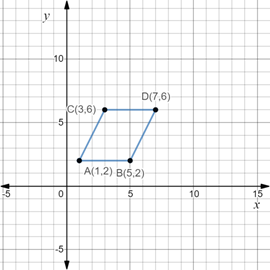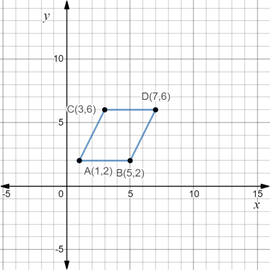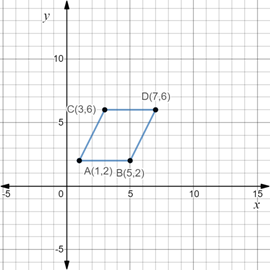# The area of the parallelogram and its figure on coordinate plane.### Precalculus: Mathematics for Calcu...

6th Edition
Stewart + 5 others
Publisher: Cengage Learning
ISBN: 9780840068071### Precalculus: Mathematics for Calcu...

6th Edition
Stewart + 5 others
Publisher: Cengage Learning
ISBN: 9780840068071

#### Solutions

Chapter 1.8, Problem 20E
To determine

## To calculate: The area of the parallelogram and its figure on coordinate plane.

Expert Solution

The area of the parallelogram is 16 sq. units and its figure is,### Explanation of Solution

Given information:

The four vertices of the parallelogram A(1,2),B(5,2),C(3,6) and D(7,6) .

Formula used:

Area of a parallelogram is the product of its base and height. So, if b is the base and h is its height, area of parallelogram is calculated as,

A=bh

Calculation:

Consider the given four vertices of the parallelogram A(1,2),B(5,2),C(3,6) and D(7,6) .

Observe that all the points lie in the first quadrant of the coordinate plane, so, plot the points in first quadrant of the coordinate plane and join the vertices to form the parallelogram as,In the above figure, it is observed that the base of parallelogram is 4 units and its height is 4 units.

Recall that the area of a parallelogram is the product of its base and height. So, if b is the base and h is its height, area of parallelogram is calculated as,

A=bh

Apply it,

A=bh=44=16 sq. units

Thus, area of the parallelogram is 16 sq. units and its figure is,### Have a homework question?

Subscribe to bartleby learn! Ask subject matter experts 30 homework questions each month. Plus, you’ll have access to millions of step-by-step textbook answers!